Function Repository Resource:

# IntraclassCorrelation

Find the intraclass correlation coefficient (ICC) of a list of lists

Contributed by: Katja Della Libera
 ResourceFunction["IntraclassCorrelation"][lst] returns the intraclass correlation coefficient (ICC) of the list of lists lst.

## Details

The intraclass correlation is a measure of repeatability when a number of measurements are performed over time or by different observers.
The ICC r can be calculated as the group-level variance VG (variance in each of the subgroups) over the sum of group- and data-level (residual) variance VR. It is given by the formula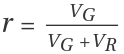.
Low intraclass correlation indicates that the value measured within each group is not significantly correlated and therefore has low repeatability.

## Examples

### Basic Examples (1)

Calculate the repeatability of a list of three different quantities, where for each we have 4 measurements:

 In:=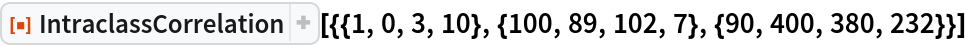Out=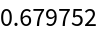### Scope (2)

An example of a data set with high intraclass correlation:

 In:=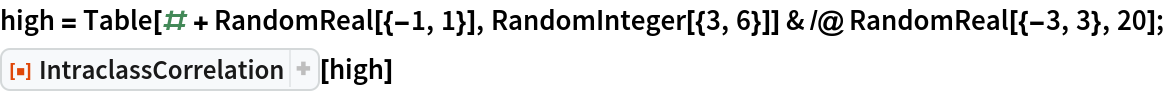Out=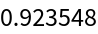A plot of the highly repeatable values:

 In:=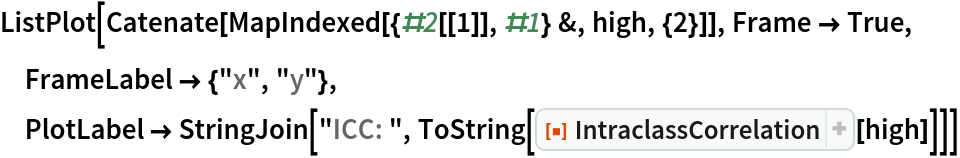Out=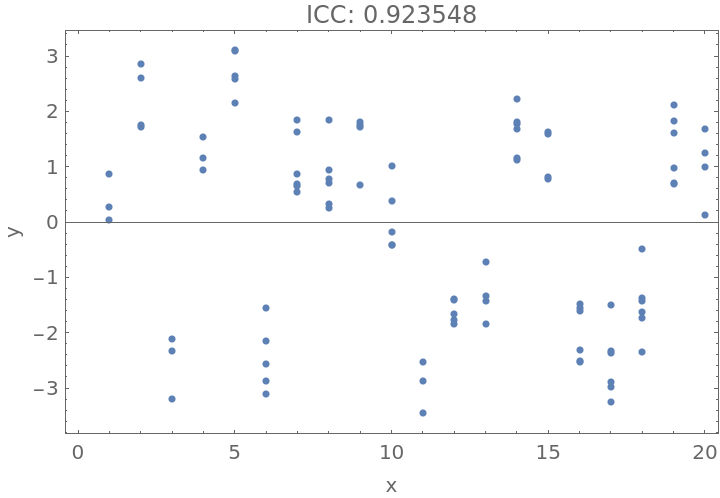An example of data with low intraclass correlation:

 In:=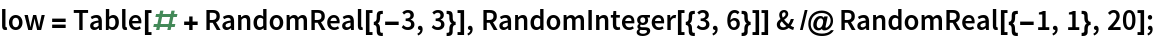In:=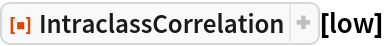Out=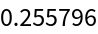A plot of the data:

 In:=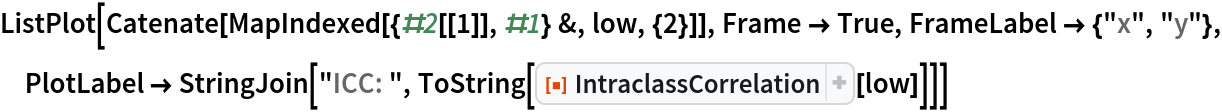Out=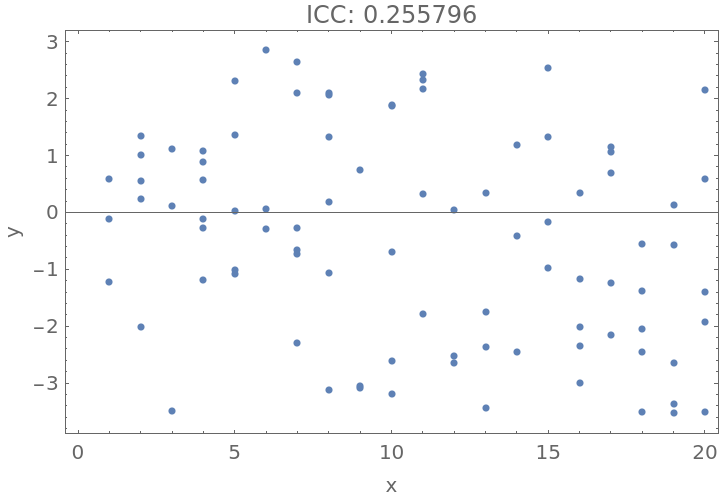## Publisher

Katja Della Libera

## Version History

• 1.0.0 – 20 January 2021